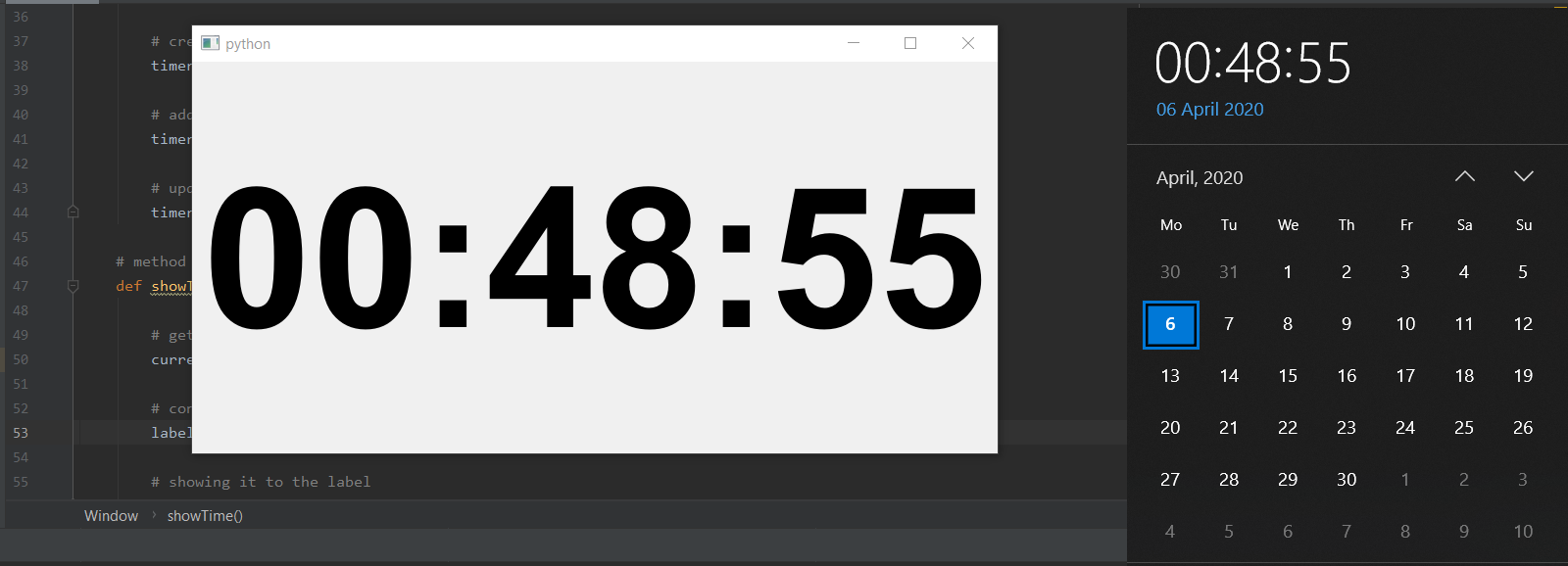Open in App
Not now

# PyQt5 – Create a digital clock

• Last Updated : 06 Jan, 2023

In this article we will see how to create a digital clock using PyQt5, this digital clock will basically tells the current time in the 24 hour format.

In order to create a digital clock we have to do the following:

1. Create a vertical layout
2. Create label to show the current time and put it in the layout and align it to the center.
3. Create a QTimer object.
4. Add action to the QTimer object such that after every 1sec action method get called.
5. Inside the action method get the current time and show that time with the help of label.

Below is the implementation:

## Python3

 `# importing required librarie` `import` `sys` `from` `PyQt5.QtWidgets ``import` `QApplication, QWidget` `from` `PyQt5.QtWidgets ``import` `QVBoxLayout, QLabel` `from` `PyQt5.QtGui ``import` `QFont` `from` `PyQt5.QtCore ``import` `QTimer, QTime, Qt`     `class` `Window(QWidget):`   `    ``def` `__init__(``self``):` `        ``super``().__init__()`   `        ``# setting geometry of main window` `        ``self``.setGeometry(``100``, ``100``, ``800``, ``400``)`   `        ``# creating a vertical layout` `        ``layout ``=` `QVBoxLayout()`   `        ``# creating font object` `        ``font ``=` `QFont(``'Arial'``, ``120``, QFont.Bold)`   `        ``# creating a label object` `        ``self``.label ``=` `QLabel()`   `        ``# setting center alignment to the label` `        ``self``.label.setAlignment(Qt.AlignCenter)`   `        ``# setting font to the label` `        ``self``.label.setFont(font)`   `        ``# adding label to the layout` `        ``layout.addWidget(``self``.label)`   `        ``# setting the layout to main window` `        ``self``.setLayout(layout)`   `        ``# creating a timer object` `        ``timer ``=` `QTimer(``self``)`   `        ``# adding action to timer` `        ``timer.timeout.connect(``self``.showTime)`   `        ``# update the timer every second` `        ``timer.start(``1000``)`   `    ``# method called by timer` `    ``def` `showTime(``self``):`   `        ``# getting current time` `        ``current_time ``=` `QTime.currentTime()`   `        ``# converting QTime object to string` `        ``label_time ``=` `current_time.toString(``'hh:mm:ss'``)`   `        ``# showing it to the label` `        ``self``.label.setText(label_time)`     `# create pyqt5 app` `App ``=` `QApplication(sys.argv)`   `# create the instance of our Window` `window ``=` `Window()`   `# showing all the widgets` `window.show()`   `# start the app` `App.exit(App.exec_())`

Output :Code Explanation:

1. The code starts by importing the required libraries.
2. Then it creates a class called Window and initializes it with __init__() method.
3. Next, the code sets the geometry of the main window to 100×100 pixels and 800×400 pixels respectively.
4. It then creates a vertical layout object which is used for arranging widgets in order vertically on the screen.
5. The next step is creating font object using QFont class from PyQt5 library followed by creating a label object using QLabel class from PyQt5 library.
6. Finally, the code adds a label to the layout and sets its alignment to Qt’s align center option before setting font of label to Arial font with a size 120 points bold style.
7. The code is a simple example of how to create a window and show it.

My Personal Notes arrow_drop_up
Related Articles